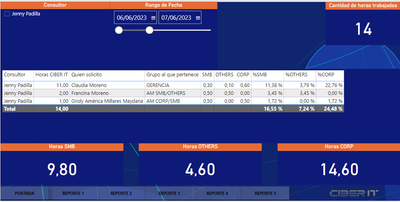cancel
Showing results for
Did you mean:Regular Visitor

## Measures and calculated columns

i need to do this simple formula (A1/A2)*A3, A1 represent the hour, A2 the sum of all the hours and A3 the value assigned to it depending on the type (SMB, OTHERS CORP) .

i already made it but using some measures and calculated columns, now the problem that i realized is that i can't use filters with the calculated columns, to get the total of hours I use a calculated column to make the operation with all the columns with the individual hours and the values of SMB, OTHERS and CORP, as you notice the general is 29 but if i filter by the date the total of hours decrease but the fields keep the value because i use a calculated column and it doesn't change.(Here using 29 hours as the total, the calculations are correct, even percentage total gives 100%)(But if i change the date the total of hours change and the calculations continue to be made under the data of the previous total, and the percentage total doesn't gives 100%)

and the fields below still the same because they are made by calculated columns so they will never change, but i need them to change, the sum of them should give the total of hours that in this case is 14 not 29)

pls someone help me.Super User

You are right - as soon as the results of a computation must be influenced by a user's interaction with your report then you need to use measures.

Start over and implement your logic with measures.